### Multiplication Chart TableMultiplication Chart in the subject mathematics is one of the crucial topics when it comes to learning and growth of the students. With the growing technologies and new structures, students now-a-days have the constant urge to constantly learn new and complex content as they grow through their education. Mathematics is known to be a complex and tough subject, so it is important that students learn in a fun and engaging way all the important list of subjects and topics. In the initial level of school and learning process Multiplication Table seems like a tough nut to crack.

Multiplication Table Worksheet is available online for students to have their regular practice session. Multiplication Chart for Kids is created in a way that can be easily understood and learnt by students of all stages. The list of Blank Multiplication Chart will enable students to learn and fill the chart online and this is an interesting way of making students learn the multiplication chart. For all the students/parents/teachers Printable Multiplication Chart is also available that is easily available for printing purposes and can be used at anytime and from anywhere. The copy of Free Printable Multiplication Table is available on the site and can be checked by anyone. It is one of the best ways to keep regular practice session for your child in a fun and engaging way.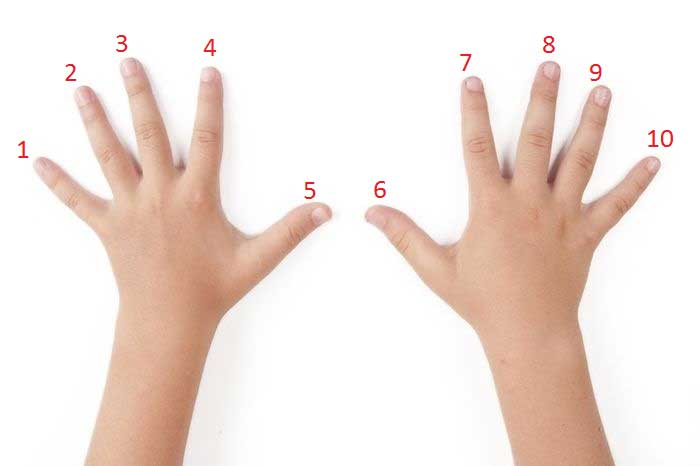We have covered various type of Multiplication Table Charts --

Learning multiplication table is an important aspect of school life and helps in future as well. The process of calculation once you grow up helps if you have a good hold of multiplication tables from the beginning. So, now that you know the availability of multiplication tables is just a click away its time that you engage your kids in a fun and interesting way of learning. Let your child have good practice sessions of multiplication tables from the beginning stages.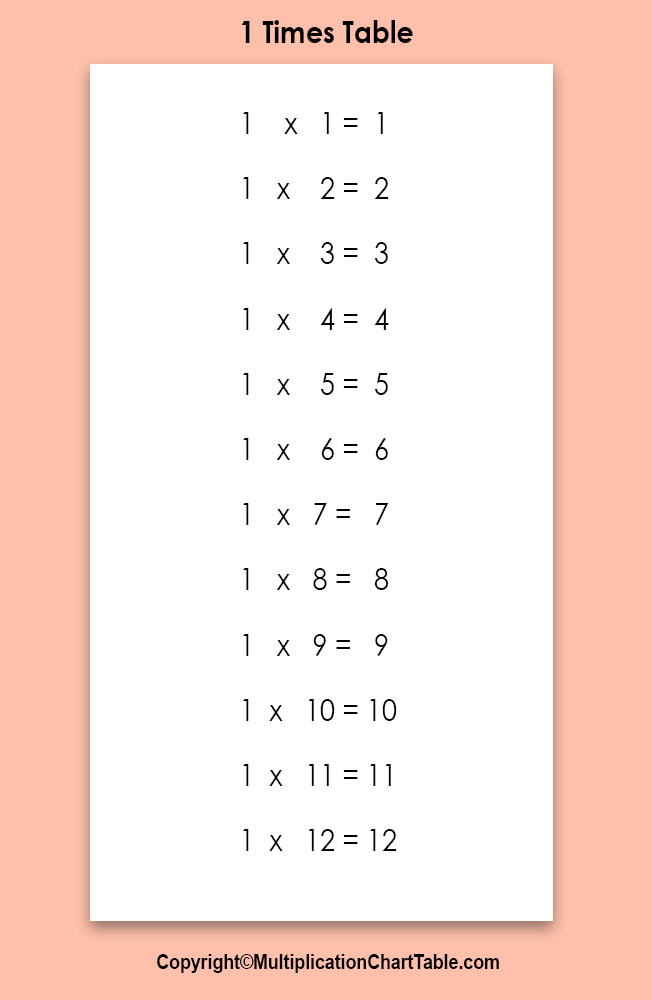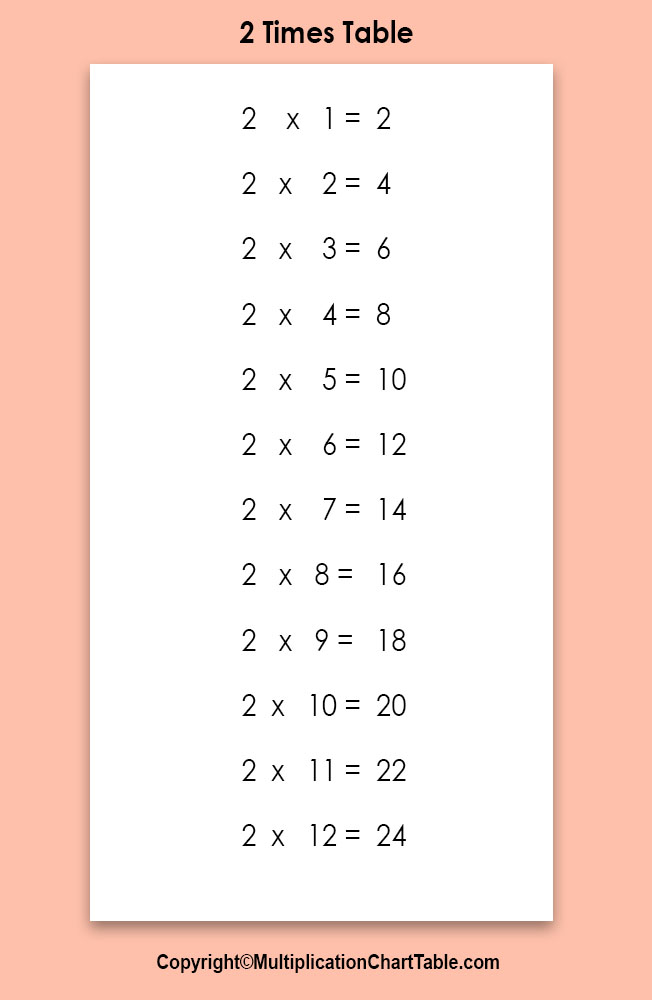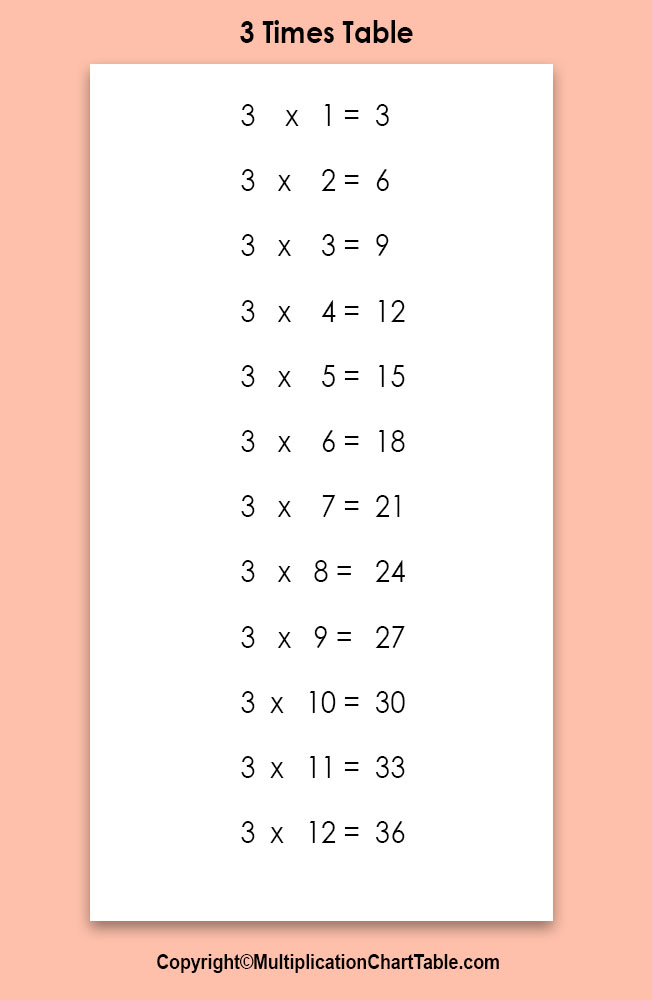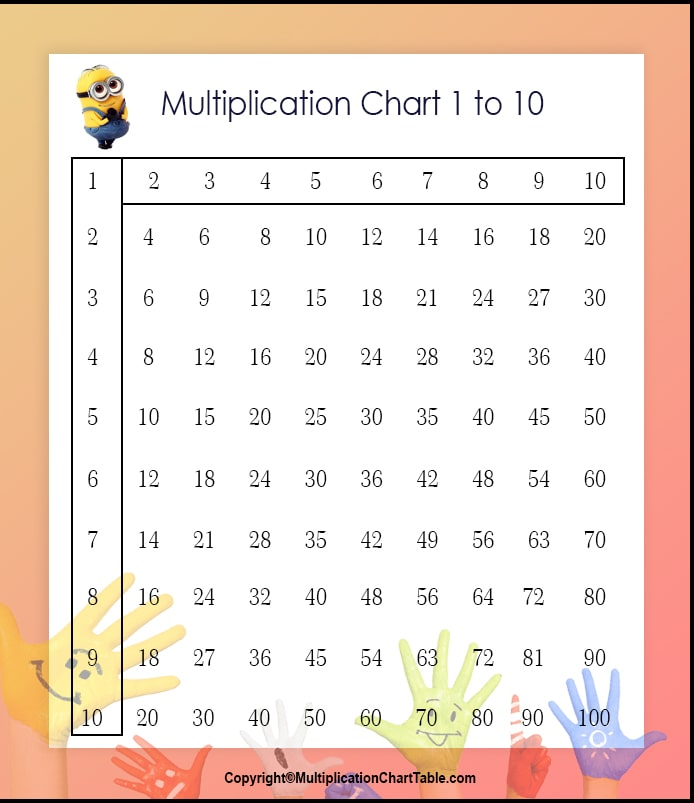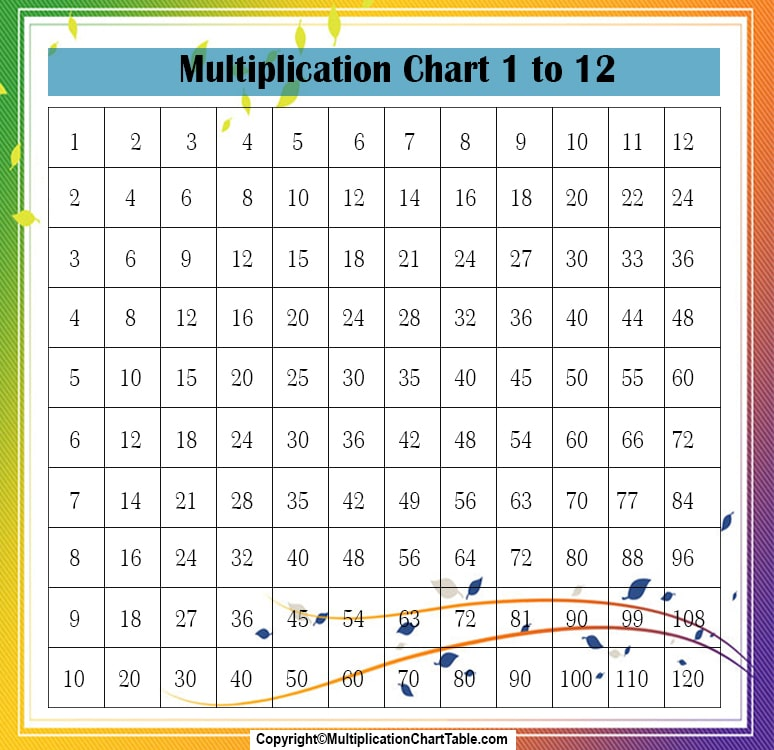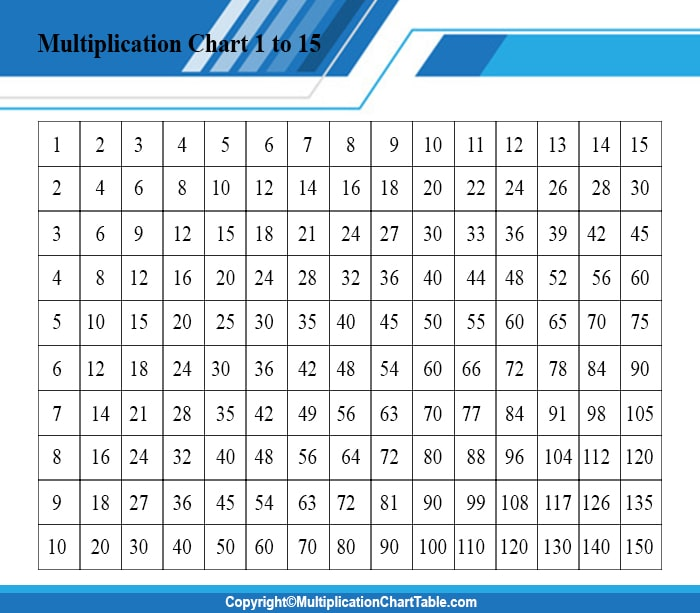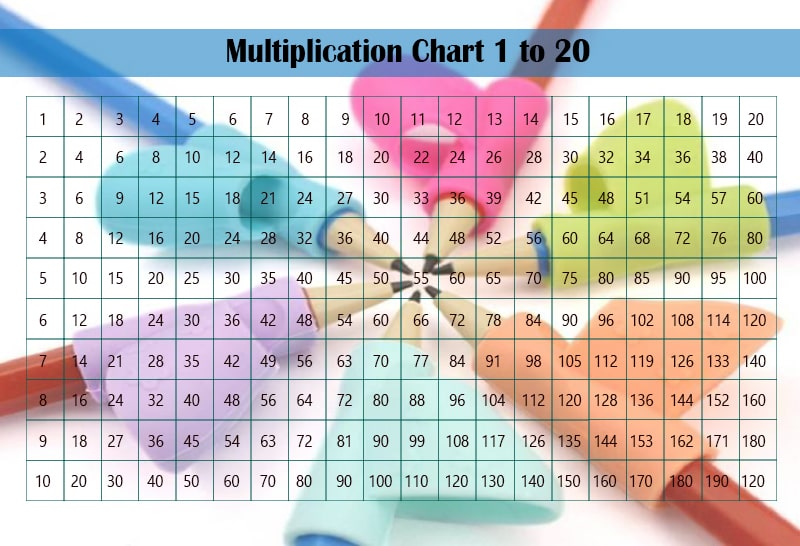## Understanding Multiplication

Multiplication is better understood when it is referred to as “group of” instead of “times”. Being a parent/teacher, you can make the students understand that in the process of multiplication they are adding together groups of numbers.

For example: 3 x 4 becomes 3 groups of 4

Or 4+4+4 = 12

Multiplication is the process of adding groups of numbers together. This is one of the easiest methods of making students understand why they’re multiplying and how it works.

## Tips to memorize 1-12 Multiplication tables

When it comes to learning multiplication tables, students take it as a tough and difficult task. How to memorize the multiplication tables is something that every student struggle with, but it is important to learn all the tables.

So, here are some easy tips that will help you in learning the 1-12 multiplication tables.

## Tips for the 1 Times table

When any number is multiplied by the number 1 it stays the same. In these equations it always means that there’s only1 group of number.

## Tips for the 2 Times table

When any number is multiplied by the number 2 it becomes doubled. You can also explain this to students by telling them that they can think of that they are adding 2 of the same number.

For eg: 4x2 is same as 4+4

## Tips for the 3 Times table

The multiplication Times table 3 doesn’t have any particular rules to remember, but there is a pattern for every 10 multiples of 3. For eg: 3,6,9,12,15 …… and so on…. The last digit of these multiples always repeat, which means that students can remember these digits to help them with the 3 multiplication table.

If we look at the next set of numbers which are 33,36,39,42,45…… and so on…. The last set of numbers i.e. 3,6,9,2… are repeated. This will help students in atleast knowing the last number.

## Tips for the 4 Times table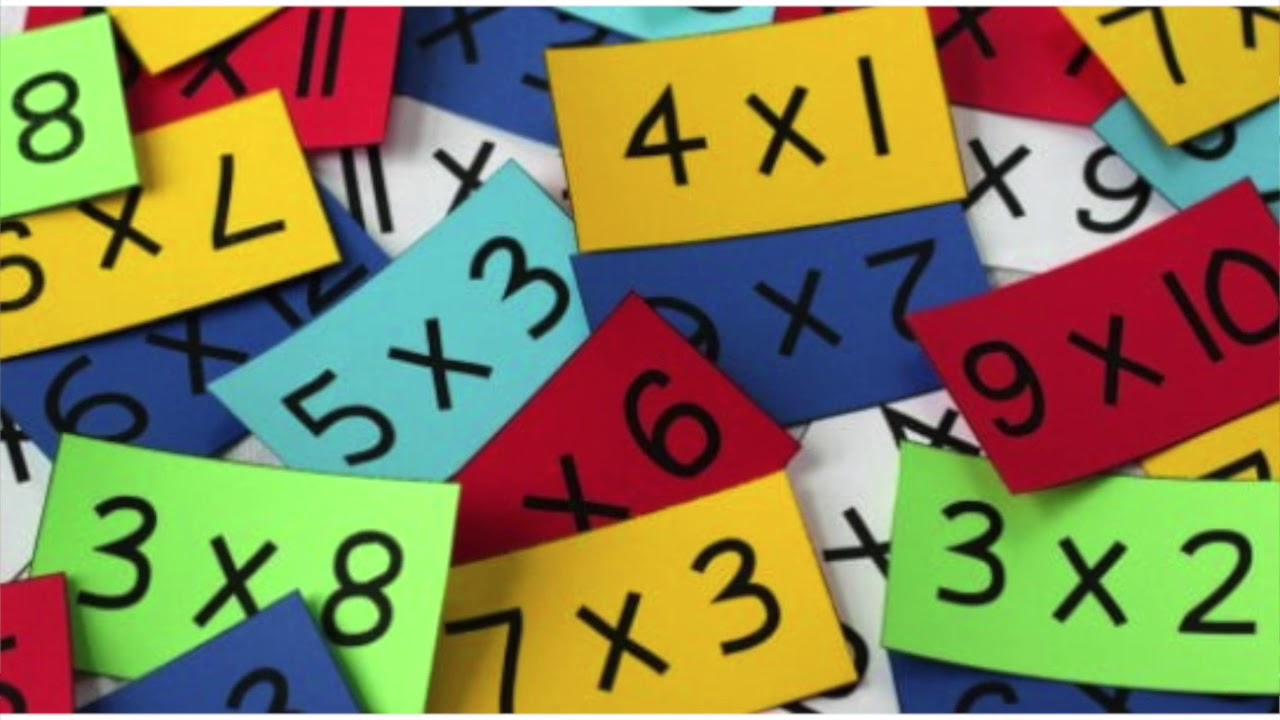In the 4 Times table, when a number is multiplied by 4 it means double it and then double it again.

For eg: 5X4 becomes 5+5 = 10 and 10+10 = 20.

## Tips for the 5 Times table

Number 5 is the easiest Times table to learn for the students. You simply have to tell the students to learn the pattern of 5,0,5,0,5,0 …..

## Tips for the 6 Times tableIn the 6 Times table, when a number is multiplied by 6, the solution always ends with the last digit of the number being multiplied.

For eg: 2x6 = 12, 74x6 =444

However, the trick is only applicable for even numbers and not for odd numbers.

## Tips for the 7 Times table

Some of the previous tips can help in remembering the 7 Times tables such as multiplying it by 2 means doubling it, multiplying by 3 means it ends in a number 1, multiplying it by 4 is like doubling it twice and any odd number multiplied by 5 will result in the end number 5.

But, for the later numbers is counting upwards for eg: 7x8 = 56, which means 5-6-7-8.

## Tips for the 8 Times table

Well, 8 Times table have the easiest tip, which is double, double, and then double again.

## Tips for the 9 Times table

The 9 Times table have two tips and first one is like multiplying by the other number by 10 and then subtracting the other number.

For eg: 9x5=45, which mean 10x5=50 (50-5=45)

The 2nd tip works only for the first ten multiples which is, every time students increase what is multiplied by 9 the tens solution column increases by 1 and the ones column decreases by 1.

## Tips for the 10 Times table

This is the easiest Times table with no tip, students just have to add 0 to every number multiplied by 10.

## Tips for the 11 Times table

11 Times table is also easy to learn students just have to repeat the digit they are multiplying by 11.

## Tips for the 12 Times table

The tip to learn 12 Times table students will have to use two Times table 10 and 2, then add them together.

For eg: 12x6 = 72

(10x6 = 60) + (2x6)

60+12 = 72

So, these were the tips that will help students in remembering the multiplication tables in an easy way.